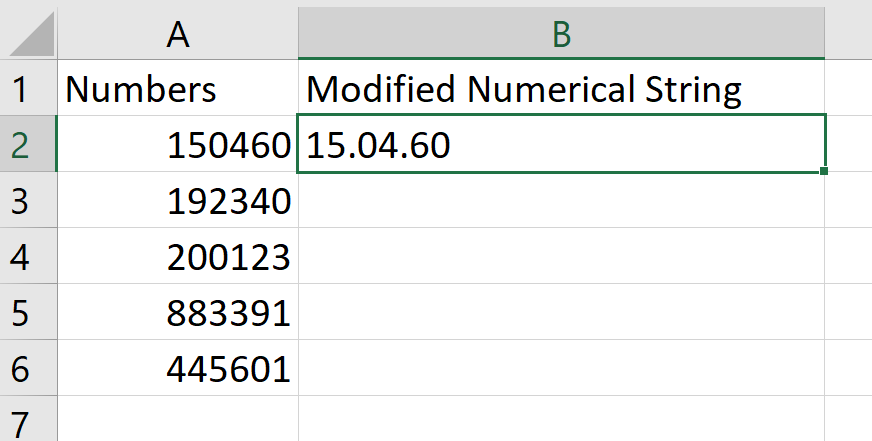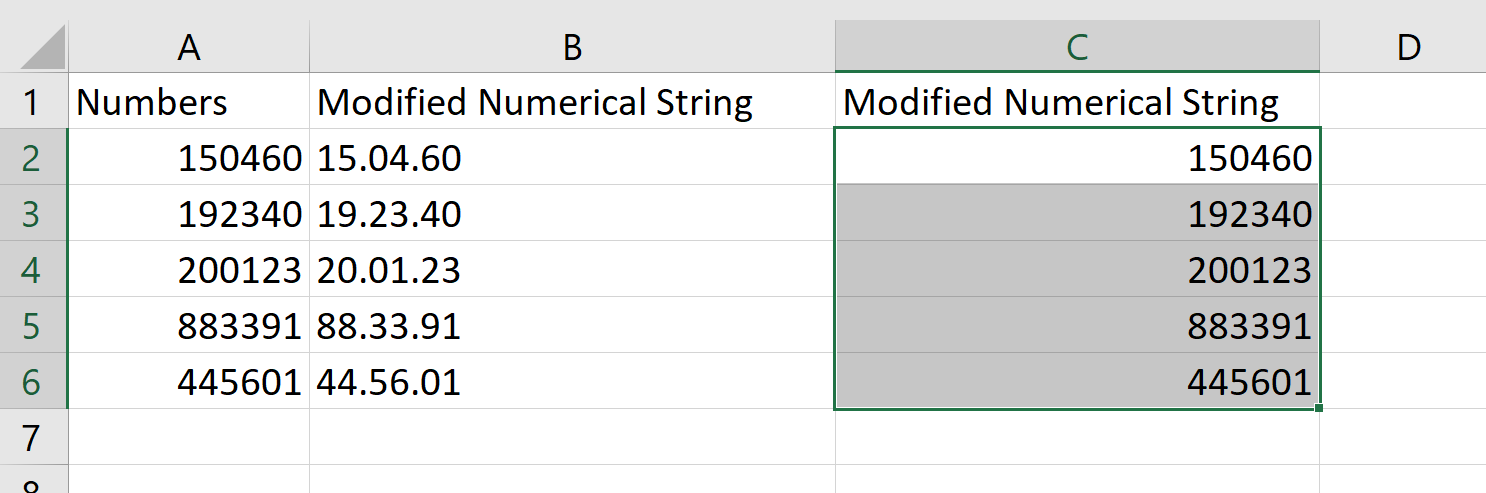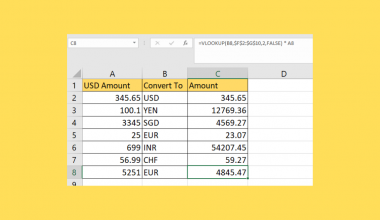# How to Insert a Dot Between Numbers in Excel

Both the REPLACE function and custom formatting options are helpful when you need to insert dots between numbers in Excel.

With the REPLACE function, we can insert characters in a specific location in a string. Excel also offers a powerful number formatting tool to help us separate numbers by dots.

Let’s take a look at a quick example where we might need to insert one or more dots between numbers in Excel.

Suppose you have a list of items in an inventory sheet. Each unique item is indicated by a 10-digit number such as ‘0003484112’. The first four digits indicate the item category, the next three digits refer to the manufacturer, and the last three digits are unique to the item itself.

To make it easier to read the serial numbers, you’ve decided to add dots between each section. How can you do this in Excel?

With the `REPLACE` function, we can add any arbitrary character to a string. For example, `REPLACE(“1234567890”;5;0;".") `returns the string ‘1234.567890’ where a dot is placed as the fifth character. We can use multiple `REPLACE` functions in a single formula to keep adding characters in the necessary places.

Users can also take advantage of Excel’s custom number formatting options. For example, if we set a cell to have the formatting ‘00”.”00”.”00’, a dot will be placed after the second and fourth digit.

Now that we know what methods we can use to insert dots between numbers, let’s look at an actual sample spreadsheet that applies these methods.

## A Real Example of Inserting Dot Between Numbers in Excel

Let’s take a look at a real example of an Excel spreadsheet that successfully inserts dots between numbers.

In the example below, we’ve used custom formatting to add a dot after every second and fourth digit. The values in column B are derived from an Excel formula, while the values in column C are duplicates of the original numbers with additional formatting options.To get the values in Column B, we just need to use the following formula:

`=REPLACE(REPLACE(A2;3;0;".");6;0;".")`

The `REPLACE` function replaces part of a given text string with a different text string. The user can specify how many characters from a given position will be replaced.

In this particular use case, we do not need to replace any characters. Instead, we use `REPLACE` to insert a character into a given place. First, we use the formula `REPLACE(A2,3,0,".") `to add a period after the second character. The ‘0’ in the third argument indicates that no characters will be replaced with the dot.

We then take the result of that formula and place it as an argument in another `REPLACE` to add the second dot after the fourth digit.

If you’re ready to try out these methods in Excel, let’s start writing them ourselves!

## How to Insert a Dot Between Numbers in Excel

This section will guide you through each step you need to insert dots between numbers in Excel. You’ll learn how we can use both Excel functions and custom number formatting to add characters into a string.

Follow these steps to learn how to use the `REPLACE` function:

1. First, select the cell that will hold our modified string. In this example, we’ll add our formula to cell B2.2. Next, we simply add the `REPLACE` formula mentioned in the last section. Make sure that the cell references are correct. Hit the Enter key to return the modified string.3. Drag down the formula to fill in the rest of the cells in the column.Alternatively, you can use custom number formatting instead of inserting dots between numbers in Excel.

First, you must figure out what format to use. For example, if you want to place a dot after every number in a four-digit string, use the string ‘0”.”0”.”0”.”0’ when indicating your custom format.

1. Select the numbers you want to modify. In this example, we’ve created a copy of our original values in column A.2. Next, open up the Format Cells dialog box by using the shortcut Ctrl + 1. For the Category option, select Custom. In the textbox under Type, place the desired custom formatting option. In the example below, we’ve used a formatting option that places a dot after the second and fourth numbers.3. Click on the OK button to apply the changes to the selected cells.This step-by-step guide should be useful if you ever need to insert dots between numbers in Excel. Our guide shows how easy it is to use both the `REPLACE` function and custom number formatting to add dots in specific places in a numerical string.

The `REPLACE` function is just one example of a text function you can use in Excel. With so many other Excel functions available, you can certainly find a few that can help improve your workflow in Excel.

Are you interested in learning more about what Excel can do? Subscribe to our newsletter to find out about the latest Excel guides and tutorials from us.### Get emails from us about Excel.

Our goal this year is to create lots of rich, bite-sized tutorials for Excel users like you. If you liked this one, you'd love what we are working on! Readers receive ✨ early access ✨ to new content.

##### You May Also Like## How to Divide a Range of Cells by a Number in Excel

This guide will show you how you can divide a range of cells by a number in Excel.…## How to Use MATCH Function in Excel

The MATCH function in Excel is useful when you need to give the relative position of an item…## How to Protect Sheet but Allow Data Entry in Excel

This guide will explain how you can protect a sheet but still allow data entry in Excel. Excel’s…## How to Do Currency Conversion using VLOOKUP in Excel

This guide will explain how we can perform currency conversion using the VLOOKUP function in Excel. The VLOOKUP…